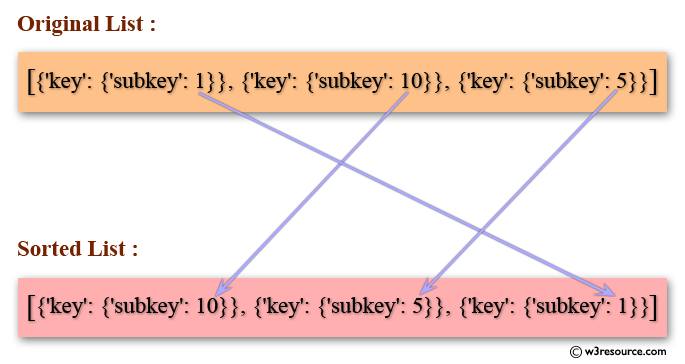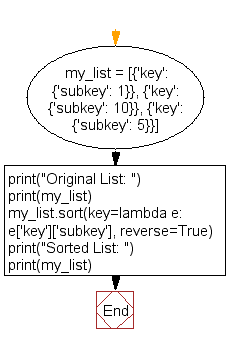﻿ Python: Sort a list of nested dictionaries - w3resource# Python: Sort a list of nested dictionaries

## Python List: Exercise - 50 with Solution

Write a Python program to sort a list of nested dictionaries.Sample Solution:-

Python Code:

``````my_list = [{'key': {'subkey': 1}}, {'key': {'subkey': 10}}, {'key': {'subkey': 5}}]
print("Original List: ")
print(my_list)
my_list.sort(key=lambda e: e['key']['subkey'], reverse=True)
print("Sorted List: ")
print(my_list)
```
```

Sample Output:

```Original List:
[{'key': {'subkey': 1}}, {'key': {'subkey': 10}}, {'key': {'subkey': 5}}]
Sorted List:
[{'key': {'subkey': 10}}, {'key': {'subkey': 5}}, {'key': {'subkey': 1}}]
```

Flowchart:## Visualize Python code execution:

The following tool visualize what the computer is doing step-by-step as it executes the said program:

Python Code Editor:

Have another way to solve this solution? Contribute your code (and comments) through Disqus.

What is the difficulty level of this exercise?

Test your Python skills with w3resource's quiz

﻿

## Python: Tips of the Day

Checks if the given number falls within the given range.

Example:

```def tips_range(n, start, end = 0):
return start <= n <= end if end >= start else end <= n <= start
print(tips_range(2, 4, 6))
print(tips_range(4, 8))
print(tips_range(1, 3, 5))
print(tips_range(1, 3))
```

Output:

```False
True
False
True
```